# Surface

One of the basic concepts in geometry. The definitions of a surface in various fields of geometry differ substantially.

In elementary geometry, one considers planes, multi-faced surfaces, as well as certain curved surfaces (for example, spheres). Each curved surface is defined in a special way, very often as a set of points or lines. The general concept of surface is only explained, not defined, in elementary geometry: One says that a surface is the boundary of a body, or the trace of a moving line, etc.

In analytic and algebraic geometry, a surface is considered as a set of points the coordinates of which satisfy equations of a particular form (see, for example, Surface of the second order; Algebraic surface).

In three-dimensional Euclidean space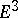, a surface is defined by means of the concept of a surface patch — a homeomorphic image of a square in. A surface is understood to be a connected set which is the union of surface patches (for example, a sphere is the union of two hemispheres, which are surface patches).

Usually, a surface is specified inby a vector function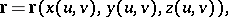where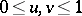, while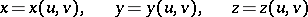are functions of parametersandthat satisfy certain regularity conditions, for example, the condition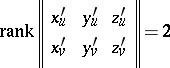From the point of view of topology, a surface is a two-dimensional manifold.